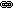Mass–energy equivalence | E=MC2
 In physics, mass–energy equivalence is the concept that any mass has an associated energy and vice versa. In special relativity this relationship is expressed using the mass–energy equivalence formulaE = mc2 Where E = energy, m = mass, c = the speed of light in a vacuum (celeritas), and the superscript 2 indicates the squaring of the c. Guess, what is E = MHT Posted By Edvin Teo At 11:21 AM • Comments (0)